# MCAT Physical : Potential Energy (Gravitational and Spring)

## Example Questions

2 Next →

### Example Question #37 : Work, Energy, And Power

A 2kg box slides down a ramp a distance of two meters before it reaches the ground. The ramp has an angle of 30o. The coefficient of kinetic friction for the ramp is 0.1.

What is the gravitational potential energy of the box relative to the ground when at the top of the ramp?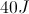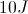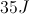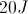Explanation:

Gravitational potential energy is determined using the equation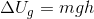, withbeing the mass of the object,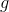being the gravitational acceleration, andbeing the height of the object relative to the ground. Because we know the length of the ramp and the angle of the ramp, we can solve for the box's height above the ground.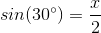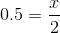Now that we have the height, we simply plug the given values into the equation.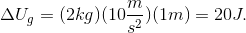### Example Question #38 : Work, Energy, And Power

A spring is used to launch a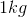ball straight up to a maximum height of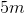. The spring constant of the spring is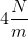.

What was the displacement of the spring required to launch the ball?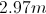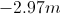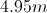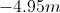Explanation:

We can use conservation of energy to calculate the displacement. The spring must be displaced so that its potential energy is equal to the gravitational potential energy of the ball at its maximum height.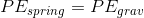Substituting in the expressions for potential energy of the spring and gravitational potential energy, we can start to set up an equation using the given variables.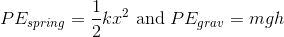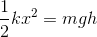We are given the spring constant, the mass, and the maximum height. Using these values, and the acceleration for gravity, we can solve for the spring displacement.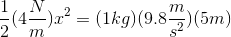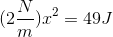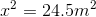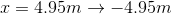Note that, since we are taking the square root, the displacement answer could be either positive or negative. Since the spring is being compressed to launch the ball (rather than stretched), the displacement should be negative.

### Example Question #39 : Work, Energy, And Power

What is the potential energy of a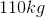block of cement being held by a crane at a height of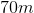?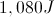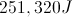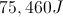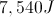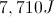Explanation:

Our formula for gravitational potential energy is: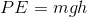is mass in kilograms,is the acceleration of gravity, andis the height in meters. We know each of these values, allowing us to solve for the energy by multiplication.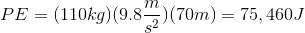### Example Question #40 : Work, Energy, And Power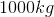pyramid stone is raised to a height of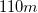. What is the potential energy of the system?

It depends if the stone is raised vertically or along an incline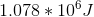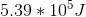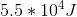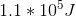Explanation:

No matter how you raise the stone (all at once, in multiple vertical steps, or along a ramp) the final potential energy is the same. Gravitational potential energy of a given object is solely dependent on height.Use the given mass, the acceleration of gravity, and the final height to calculate the potential energy of the system.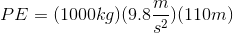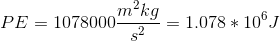Since work is equal to the change in potential energy of a system, this value also gives the total work done to raise the stone.

2 Next →